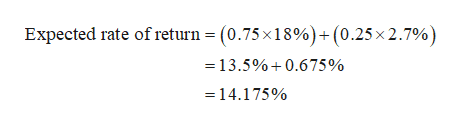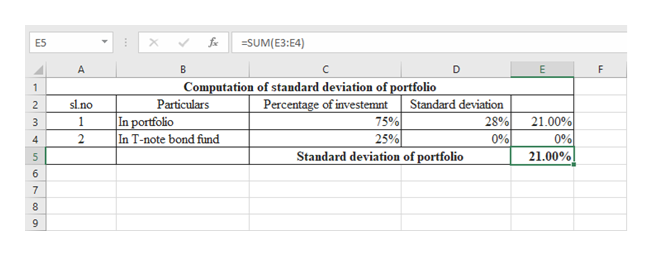Assume that you manage a risky portfolio with an expected return of18% and a standard deviation of 28%.  The T-note rate is 2.7%.  If your client chooses to invest 75% of a portfolio in your fund and 25% in a T-note bond fund.a. What is the expected return of your client's portfolio?b. What is the standard deviation of your client's prtfolio?

Question

Assume that you manage a risky portfolio with an expected return of18% and a standard deviation of 28%.  The T-note rate is 2.7%.  If your client chooses to invest 75% of a portfolio in your fund and 25% in a T-note bond fund.

a. What is the expected return of your client's portfolio?

b. What is the standard deviation of your client's prtfolio?

Step 1

a.

The expected return of portfolio can be computed by the following equation:

Step 2

Therefore, the computation of expected return of portfolio is as follows:

Therefore, the expected rate of return of the client’s portfolio is 14.175%.help_outlineImage TranscriptioncloseExpected rate of return = (0.75x18%)+(0.25x 2.7%) =13.5%0.6 75 % 14.175% fullscreen
Step 3

b.

Since the standard deviation for the T-note bond fund is zero, the computation of standard deviation of the client’s p...help_outlineImage TranscriptioncloseSUM(E3:E4) E5 E A C D F Computation of standard deviation of portfolio Percentage of investemnt 75% 25% 1 Particulars In portfolio In T-note bond fund slno Standard deviation 2 28% 21.00% 0% 1 3 0% 2 4 Standard deviation of portfolio 21.00% 5 6 7 8 fullscreen

Want to see the full answer?

See Solution

Want to see this answer and more?

Our solutions are written by experts, many with advanced degrees, and available 24/7

See Solution
Tagged in